Circle TerminologyPage 1

WATCH ALL SLIDES

Slide 1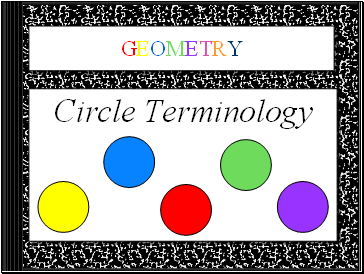GEOMETRY

Circle Terminology

Slide 2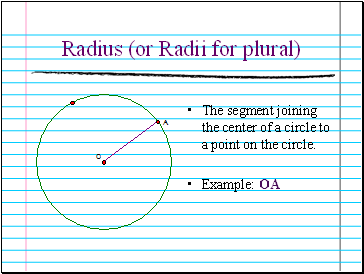The segment joining the center of a circle to a point on the circle.

Example: OA

Slide 3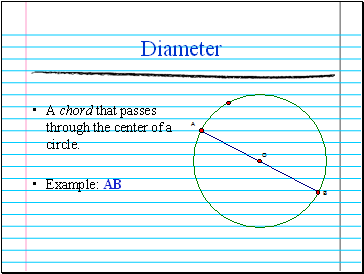Diameter

A chord that passes through the center of a circle.

Example: AB

Slide 4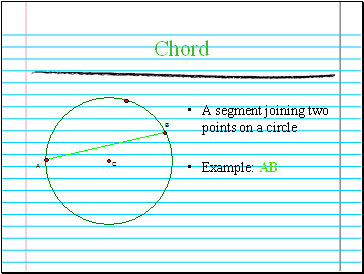Chord

A segment joining two points on a circle

Example: AB

Slide 5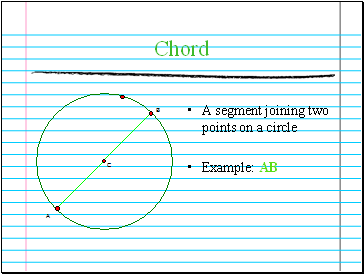Chord

A segment joining two points on a circle

Example: AB

Slide 6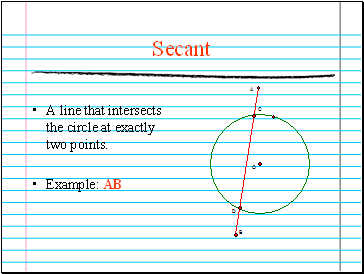Secant

A line that intersects the circle at exactly two points.

Example: AB

Slide 7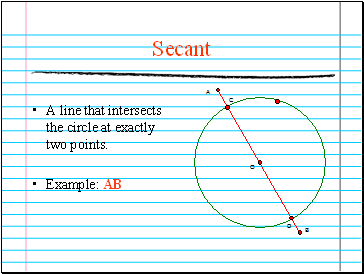Secant

A line that intersects the circle at exactly two points.

Example: AB

Slide 8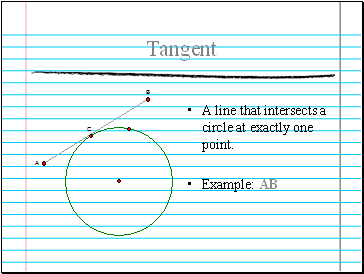Tangent

A line that intersects a circle at exactly one point.

Example: AB

Slide 9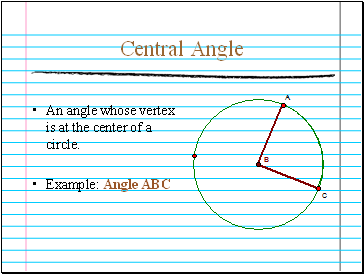Central Angle

An angle whose vertex is at the center of a circle.

Example: Angle ABC

Slide 10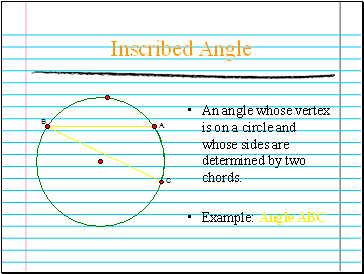Inscribed Angle

An angle whose vertex is on a circle and whose sides are determined by two chords.

Example: Angle ABC

Slide 11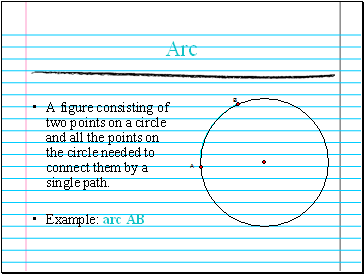Arc

A figure consisting of two points on a circle and all the points on the circle needed to connect them by a single path.

Example: arc AB

Slide 12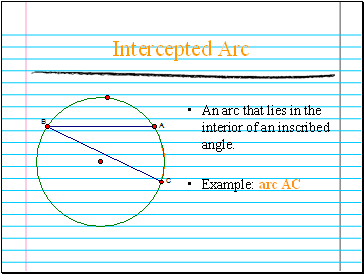Intercepted Arc

An arc that lies in the interior of an inscribed angle.

Example: arc AC

Slide 13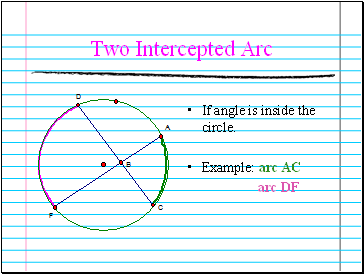Two Intercepted Arc

If angle is inside the circle.

Example: arc AC

arc DF

Slide 14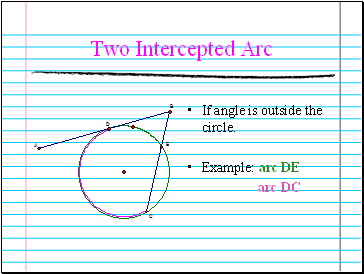If angle is outside the circle.

Example: arc DE

arc DC

Two Intercepted Arc

Slide 15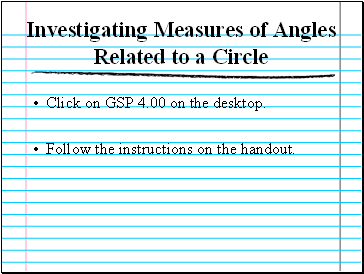Investigating Measures of Angles Related to a Circle

Click on GSP 4.00 on the desktop.

Follow the instructions on the handout.

Go to page:
1  2